# Retro (Past 13 Years) IIT - JEE Advance (Hydrocarbons)

## 15 Questions MCQ Test Chemistry for JEE Advanced | Retro (Past 13 Years) IIT - JEE Advance (Hydrocarbons)

Description
Attempt Retro (Past 13 Years) IIT - JEE Advance (Hydrocarbons) | 15 questions in 30 minutes | Mock test for JEE preparation | Free important questions MCQ to study Chemistry for JEE Advanced for JEE Exam | Download free PDF with solutions
QUESTION: 1

### Isomers of hexane, based on their branching, can be divided into three distinct classes as shown in the figure.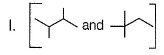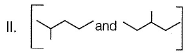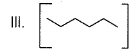Q. The correct order of their boiling point is  (2014 Adv., Only One Option Correct Type)

Solution:

III > II > I
More the branching in an alkane, lesser will be the surface area, lesser will be the boiling point.

QUESTION: 2

Solution:
QUESTION: 3

### Passage for Q. Nos. (3-4) An acyclic hydrocarbon P, having molecular formula C6H10, gave acetone as the only organic product through the following sequence of reactions, in which Q is an intermediate organic compound.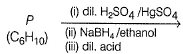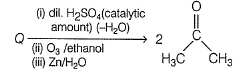Q. The structure of compound P is

Solution:
QUESTION: 4

An acyclic hydrocarbon P, having molecular formula C6H10, gave acetone as the only organic product through the following sequence of reactions, in which Q is an intermediate organic compound.Q. The structure of the compound Q is

Solution:
*Answer can only contain numeric values
QUESTION: 5

The maximum number of isomers (in cluding stereoisomers) that are possible on mono-chlorination of the following compound, is

CH3CH2CH (CH3)CH2CH2

(2011, Integer Type)

Solution:
QUESTION: 6

The synthesis of 3 -octyne is achieved by adding a bromoalkane into a mixture of sodium amide and an alkyne. The bromoalkane and alkyne respectively are

(2010, Only One Option Correct Type)

Solution:
*Answer can only contain numeric values
QUESTION: 7

The total number of cyclic isomers possible for a hydrocarbon with the molecular formula C4H6 is

(2010, Integer Type)

Solution:
QUESTION: 8

Passage for Q. N os. (8-9)

Schemes 1 and 2 describe sequential transformation of alkynes M and N. Consider only the major products formed in each step for both schemes.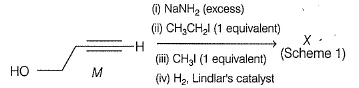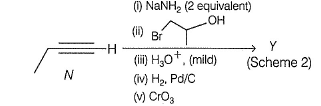Q. The product X is

(2010, Comprehension Type)

Solution:
QUESTION: 9

Schemes 1 and 2 describe sequential transformation of alkynes M and N. Consider only the major products formed in each step for both schemes.Q. The correct statement with respect to product Y is

Solution:
QUESTION: 10

The number of stereoisomers obtained by bromination of trans-2-butene is

(2007, Only One Option Correct Type)

Solution:
QUESTION: 11

The reagent(s) for the following conversion,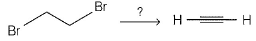is/are

(2007, Only One Option Correct Type)

Solution:
QUESTION: 12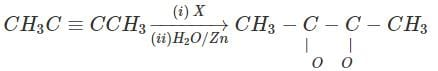X in the above reaction is

[CPMT 1985; MP PET 1997; Roorkee Qualifying 1998; DPMT 2001]

Solution: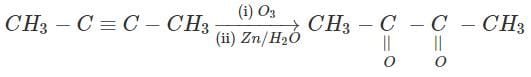QUESTION: 13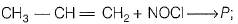Identify the adduct.

(2006, Only One Option Correct Type)

Solution:
QUESTION: 14

Cyclohexene is best prepared from cyclohexanol by which of the following ?

(2005, Only One Option Correct Type)

Solution:
QUESTION: 15

1 -bromo-3-chlorocyclobutane when treated with two equivalents of Na, in the presence of ether which of the following will be formed?

(2005, Only One Option Correct Type)

Solution:Use Code STAYHOME200 and get INR 200 additional OFF Use Coupon Code